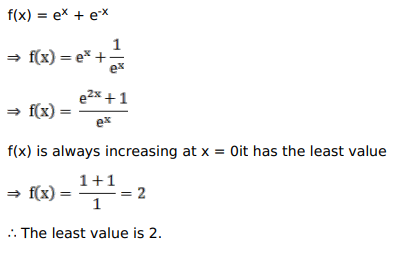# Solve this following

Question:

Mark $(\sqrt{)}$ against the correct answer in the following:

The least value of $f(x)=\left(e^{x}+e^{-x}\right)$ is

A. $-2$

B. 0

C. 2

D. none of these

Solution: